# What Is An Even Function? (5 Common Questions Answered)

Functions are useful throughout mathematics, and even functions have certain special properties that make them easier to work with.

So, what is an even function?  Algebraically, an even function f(x) is one where f(-x) = f(x) for all x values in the function’s domain.  Visually, an even function f(x) has symmetry about the y-axis (that is, the graph looks like mirror images on the left and right, reflected across the line x = 0).

Of course, there are many ways to identify even functions and use their properties.

Let’s begin.

## What Is An Even Function?

An even function is one that satisfies the equation f(-x) = f(x) for every value of x in the domain of the function. This means that it will have repeating y values.

But what does an even function look like?  Well, from a visual standpoint, an even function has symmetry from left to right.

If you cut the graph in half vertically at the line x = 0, you would get mirror images on the left and right sides.  See the example below, which is the graph of the quadratic function f(x) = -x2: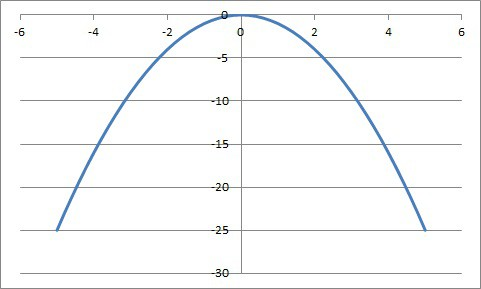The graph of the function f(x) = -x2:is a parabola that is even. There is symmetry about the y axis (the vertical line x = 0), since we have mirror images to the left and right of that line.

Here are some types of functions that are even:

• Constant Functions – also called horizontal lines, these functions have the form f(x) = c, where c is any real number (a constant).
• Quadratic Function with b = 0 – this specific type of parabola has the form f(x) = ax2 + c, where a and c are real numbers (except a is not zero).
• Cosine Function – these functions have the form f(x) = Acos(x) + B, where A and B are real numbers (except A is not zero).

Polynomial functions with only even exponents are also even functions.

Let’s look at some specific examples of even functions, along with some functions that are not even.

### Example 1: Even Function (Constant)

Take the function f(x) = 5.  This function always has a value of 5, so it is certainly true that f(-x) = f(x) for every value of x.

You can see the symmetry from left to right (mirror image across the vertical line x = 0) in the graph below.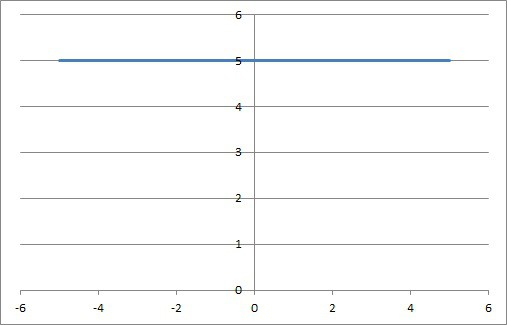The graph of the horizontal line function f(x) = 5 is even. There is symmetry about the y axis (the vertical line x = 0), since we have mirror images to the left and right of that line.

### Example 2: Even Function (Quadratic With b = 0)

Recall that a quadratic function has the form f(x) = ax2 + bx + c, where a is not zero.  If b = 0, then the middle term is “missing” (it is really zero).

Take the function f(x) = 3x2 + 5, which has a = 3, b = 0, and c = 5.  We can prove that f(-x) = f(x) as follows, just by using the function definition and the rules of exponents:

• f(x) = 3x2 + 5  [original function]
• f(-x) = 3(-x)2 + 5  [plug in –x to test for an even function]
• f(-x) = 3x2 + 5  [(-x)2 = (-x)(-x) = +x2, since the product of two negatives is positive]
• f(-x) = f(x)  [since f(x) = 3x2 + 5]

Thus, this quadratic function with b = 0 is an even function.

You can see the symmetry from left to right (mirror image across the vertical line x = 0) in the graph below.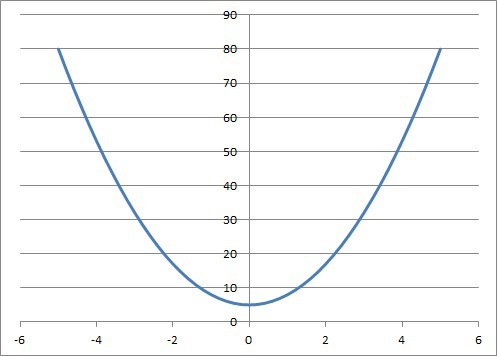The graph of the parabola f(x) = 3x2 + 5, which is an even function. There is symmetry about the y axis (the vertical line x = 0), since we have mirror images to the left and right of that line.

### Example 3: A Function That Is Not Even (Line That Is Not Horizontal)

Recall that a linear function has the form f(x) = ax + b, where a is not zero.  If a is not zero, then this function is not even.

Take the function f(x) = 2x – 7, which has a = 2 and b = -7.  We can prove that f(-x) does not equal f(x) for every x with one example: x = -1 and x = 1.

• For x = 1: f(1) = 2(1) – 7 = -5
• For x = -1: f(-1) = 2(-1) – 7 = -9

Since -5 does not equal -9, then f(1) does not equal f(-1), and f(-x) is not equal to f(x) for every x in the domain.

Thus, this line is not an even function.

You can see the lack of symmetry from left to right (across the vertical line x = 0) in the graph below.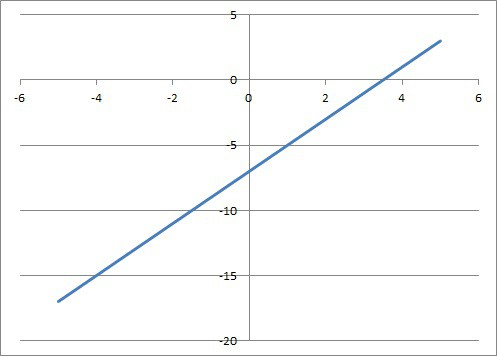The graph of the linear function f(x) = 2x – 7 is not even. There is not symmetry about the y axis (the vertical line x = 0), since we do not have mirror images to the left and right of that line.

### Example 4: A Function That Is Not Even (Cubic Polynomial)

Recall that a cubic function has the form f(x) = ax3 + bx2 + cx + d , where a is not zero.  Such a function will not be even.

Take the function f(x) = 4x3 – 7x2 + 8x + 9, which has a = 4, b = -7, c = 8, and d = 9.  We can prove that f(-x) does not equal f(x) for every x with one example: x = -1 and x = 1.

• For x = 1: f(1) = 4(1)3 – 7(1)2 + 8(1) + 9 = 4 – 7 + 8 + 9 = 14
• For x = -1: f(-11) = 4(-1)3 – 7(-1)2 + 8(-1) + 9 = -4 – 7 – 8 + 9 = -10

Since -14 does not equal -10, then f(1) does not equal f(-1), and f(-x) is not equal to f(x) for at least one x value in the domain.

Thus, this cubic function is not an even function.

You can see the lack of symmetry from left to right (across the vertical line x = 0) in the graph below.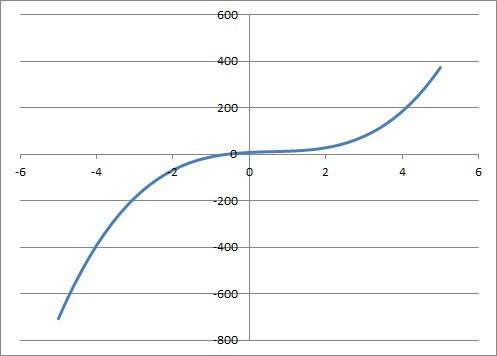The cubic function f(x) = 4x3 – 7x2 + 8x + 9 is not even. There is not symmetry about the y axis (the vertical line x = 0), since we do not have mirror images to the left and right of that line.

## How To Tell If A Function Is Even

There are a few different ways to tell that a function is even:

• Algebraically – doing this requires doing a proof by showing that f(-x) is the same as f(x).
• Graphically – doing this requires graphing the function and seeing whether the graph is symmetric about the y axis (that is, the vertical line x = 0).
• From A Table – doing this requires checking each pair of opposite x values and making sure the y values are the same.

### How To Tell If A Function Is Even Algebraically

To tell that a function is even algebraically, we need to do a proof using the given function and the definition of an even function.

For example, let’s say we have the function f(x) = x4 – x2 + 6.

To prove this function is even algebraically, we need to prove that f(-x) = f(x), since that is the definition of an even function.  So:

• f(-x) = (-x)4 – (-x)2 + 6  [using the definition of f(x) and plugging in –x]
• f(-x) = x4 – x2 + 6  [since a negative raised to an even power is positive]
• f(-x) = f(x)  [by definition, f(x) = x4 – x2 + 6]

### How To Tell If A Function Is Even From A Graph

To tell that a function is even from a graph, we need to examine the graph and see if there are mirror images to the left and right of the y axis (the vertical line x = 0).

For example, let’s say we have the quartic function f(x) = x4 – 3x2 + 2 (pictured below)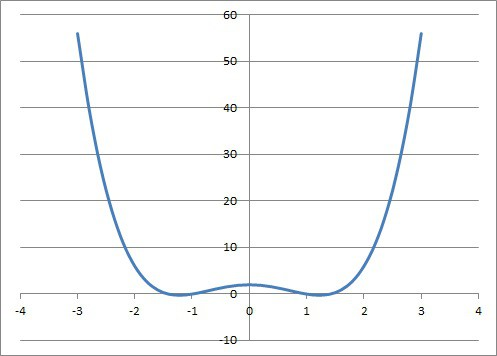The graph of the quartic function f(x) = x4 – 3x2 + 2 is even. There is symmetry about the y axis (the vertical line x = 0), since we have mirror images to the left and right of that line.

Looking at the graph, we can see that there are mirror images on the left and right of the y axis.  Thus, the function is even (or symmetric about the y axis).

### How To Tell If A Function Is Even From A Table

We can also look at a table to tell if a function is even.  We will need to see if the y values are the same for opposite x values.

For example, let’s say we have the following function table:

The y values are equal for opposite x values, since:

• f(-4) = f(4) = 8
• f(-3) = f(3) = 5
• f(-2) = f(2) = 2
• f(-1) = f(1) = 0

Since f(-x) = f(x) for every pair of x values in the table, we can infer that the graph is of an even function.

## Can An Even Function Be Negative?

An even function can be negative.  Perhaps the simplest example is the horizontal line function f(x) = -2.

This function is even and it is negative.  You can see its graph below.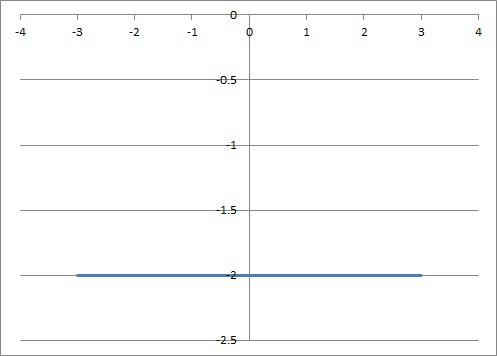The graph of the horizontal line function f(x) = -2 (a constant function), which is even and negative for every x value.

## Can An Even Function Be One-To-One (Injective)

An even function cannot be one-to-one.  The reason is that there are always two different x values (inputs) that give the same y value (output) for an even function.

Think about the definition of an even function.  It says that f(-x) = f(x) for every x value in the domain.

This tells us that two different inputs (x and –x, for x not zero) will yield the same output (that is, f(x)).

A one-to-one function has only one input that leads to a given output.

Thus, the graph of an even function will always fail the horizontal line test.

## Can An Even Function Have An Inverse?

An even function cannot have an inverse.  Remember that only an injective (one-to-one) function can have an inverse.

Since an even function is not one-to-one, it cannot have an inverse.

## Is The Sum Of Two Even Functions Always An Even Function?

The sum of two even functions is always an even function.  We can prove this in general.

Let’s say we have two even functions, f(x) and g(x).

By definition of an even function:

• f(-x) = f(x)
• g(-x) = g(x)

Let their sum be the function h(x) = f(x) + g(x).  To prove that h(x) is even, we need to show that h(-x) = h(x):

• h(-x) = f(-x) + g(-x)  [definition of h(x) with –x plugged in]
• h(-x) = f(x) + g(-x)  [since f(-x) = f(x)]
• h(-x) = f(x) + g(x)  [since g(-x) = g(x)]
• h(-x) = h(x)  [since h(x) = f(x) + g(x)]

Thus, the sum of two even functions is also an even function.

You can add up 3, 4, or as many even functions as you like, and the resulting function will be even.

## Can An Even Function Have An Inflection Point?

An even function can have an inflection point. However, not all even functions have an inflection point.

Consider the even function f(x) = x4 – 6x2.

The first derivative is f’(x) = 4x3 – 12x.

The second derivative is f’’(x) = 12x2 – 12.

The second derivative is zero when f’’(x) = 0, or:

• 12x2 – 12 = 0
• 12x2 = 12
• x2 = 1
• x = +1 or -1

Between x = -1 and x = 1, f’’(x) is negative (for example, f’’(0) = -12).

For x > 1, f’’(x) is positive (for example, f’’(2) = 36).

So, the sign of f’’(x) changes from negative to positive at x = 1.

Since the second derivative of f(x) changes signs at x = 1, then x = 1 is an inflection point on the graph of this function.

(Note: x = -1 is also an inflection point in this case).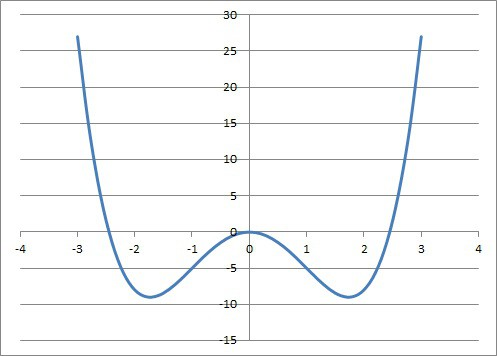The even function f(x) = x4 – 6x2 has inflection points at x = -1 and x = 1, where the concavity of the graph changes.

On the other hand, the function f(x) = x2 has no inflection point, since the second derivative is always positive (f”(x) = 2).

## Conclusion

Now you know what even functions are and what they look like.  You also know the answers to some common questions about even functions.

You might also want to read my article on odd functions.